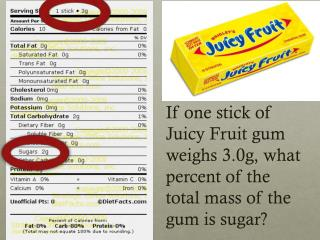Download PresentationDo Now :

# Do Now : - PowerPoint PPT PresentationDownload Presentation## Do Now :

- - - - - - - - - - - - - - - - - - - - - - - - - - - E N D - - - - - - - - - - - - - - - - - - - - - - - - - - -
##### Presentation Transcript

1. Do Now: If one stick of Juicy Fruit gum weighs 3.0g, what percent of the total mass of the gum is sugar?

2. Hydrate Lab – for tomorrow • Pre-lab • Title • Purpose • Materials • Procedure • Data table Hydrate Anhydrous

3. Hydrates • Water molecules are incorporated into the crystalline structure. Methane hydrate • Hydrates, like zinc acetate dihydrate, Zn(C2H3O2)2 * 2H2O • are commonly found in skin care products such as moisturizer, shampoo and lip balm.

4. What is the % H2O in nickel chloride dihydrate, NiCl2 * 2H2O? %H2O = = 21.76% H2O

5. part whole 24.31 g 95.21 g % = x 100 % Mg = x 100 24.305 35.453 Mg Cl 12 17 magnesium chlorine Percentage Composition (by mass...not atoms) 25.52% Mg Mg2+ Cl1- 74.48% Cl MgCl2 It is not 33% Mg and 66%Cl 1 Mg @ 24.31 g= 24.31 g 2 Cl @ 35.45 g= 70.90 g 95.21 g

6. Empirical and Molecular Formulas A pure compound always consists of the same elements combined in the same proportions by weight. Therefore, we can express molecular composition as PERCENT BY WEIGHT. Ethanol, C2H6O 52.13% C 13.15% H 34.72% O

7. Different Types of Formulas C6H6 • Molecular Formula – shows the real # of atoms in one molecule or formula unit • Empirical Formula – shows smallest whole number mole ratio **Sometimes the empirical &molecular formula can be the same • Structural Formula- molecular formula info PLUS bonding electron and atomic arrangement CH

8. Calculating Empirical formula • Percent to mass (assume 100g) • Mass to mole (molar mass) • Divide by the smallest (ratio) • Multiply til’ whole*

9. / 2.77 mol 50.04g C 5.59g H 44.37g O / 2.77 mol / 2.77 mol Empirical Formula Quantitative analysis shows that a compound contains 50.04% carbon, 5.59% hydrogen, and 44.37% oxygen. Find the empirical formula of this compound. = 1.5 C X X X 50.04% C 5.59% H 44.37% O = 4.17 mol C • *2 • *2 • *2 C3H4O2 = 5.59 mol H = 2 H = 2.77 mol O = 1 O Step 1) %  g Step 2) g  mol Step 3) mol mol Step 4) multiply til whole

10. / 0.3500 mol 66.75g Cu 10.84g P 22.41g O / 0.3500 mol / 0.3500 mol Empirical Formula Quantitative analysis shows that a compound contains 66.75% copper, 10.84% phosphorus and 22.41% oxygen. Find the empirical formula of this compound. copper (I) phosphate =3 Cu X X X 66.75% Cu 10.84 % P 22.41 % O = 1.050 mol Cu Cu3PO4 Cu3PO4 = 0.3500 mol P = 1 P = 1.401 mol O = 4 O Step 1) %  g Step 2) g  mol Step 3) mol mol

11. / 0.708 mol 32.38 g Na 22.65 g S 44.99 g O / 0.708 mol / 0.708 mol Empirical Formula Quantitative analysis shows that a compound contains 32.38% sodium, 22.65% sulfur, and 44.99% oxygen. Find the empirical formula of this compound. sodium sulfate = 2 Na X X X 32.38% Na 22.65% S 44.99% O = 1.408 mol Na Na2SO4 Na2SO4 = 0.708 mol S = 1 S = 2.812 mol O = 4 O Step 1) %  g Step 2) g  mol Step 3) mol mol